Warning: Illegal string offset 'html' in /home/hsn/public_html/forum/cache/skin_cache/cacheid_1/skin_topic.php on line 909

Log questions - Help - HSN forum# Log questions - Help

10 replies to this topic

### #1jambo david

• Gender:Male

Posted 28 March 2006 - 07:15 PM

1. Given that log10^k=2.3, write down an expression for the exact value of k

2. Solve e^3x=15

Cheers### #2SncZ

• Location:Edinburgh
• Gender:Male

Posted 28 March 2006 - 07:34 PM

1. Given that log10^k=2.3, write down an expression for the exact value of k

log10k=2.3
k=102.3

2. Solve e^3x=15

You need a calculator for this..

e3x =15
logee3x =loge15
3x(logee) =loge15

you should know that logee =1

therefore..

3x =loge15
x =(loge15) / 3 (use your calculator)

=0.9 hopefully### #3The Wedge Effect

HSN Legend

•• Members
•• 2,477 posts
• Location:Paisley
• Gender:Male

Posted 28 March 2006 - 07:40 PM

1. Given that log10^k=2.3, write down an expression for the exact value of k

2. Solve e^3x=15

CheersI'm a bit unclear on the first question. Is it asking you to find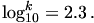or?

To find the answer for question two, you take logs of both side using the natural logarithm.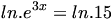Use the laws of logarithms with powers to get: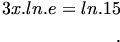ln(e) cancels to get 1 so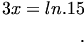In reply to SncZ, my answer would be sufficient, it's not that necessary to use a calculator, you can express it in simplified form like this, unless it specifically asks for the value to a number of decimal places.### #4SncZ

• Location:Edinburgh
• Gender:Male

Posted 28 March 2006 - 07:41 PM

wedge how do you get the 'big divide sign'

and for natural log did you just type 'ln' or is there a code for the symbol ?

### #5The Wedge Effect

HSN Legend

•• Members
•• 2,477 posts
• Location:Paisley
• Gender:Male

Posted 28 March 2006 - 07:44 PM

If you hover over the expressions, you will see the LaTeX code I used for the expressions. I just typed in 'ln' instead of using a special code, because I wasn't sure if one existed for the natural logarithm.

For the divide sign, I used the following code (example of an expression with the code is used):

CODE
[display]f(x)=\frac {x^2+2x+1}{x^2-2x+1}[/display]

The outcome of which is: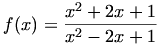### #6jambo david

• Gender:Male

Posted 28 March 2006 - 07:46 PM

1. Given that log10^k=2.3, write down an expression for the exact value of k

2. Solve e^3x=15

CheersI'm a bit unclear on the first question. Is it asking you to findor?

To find the answer for question two, you take logs of both side using the natural logarithm.Use the laws of logarithms with powers to get:ln(e) cancels to get 1 soIn reply to SncZ, my answer would be sufficient, it's not that necessary to use a calculator, you can express it in simplified form like this, unless it specifically asks for the value to a number of decimal places.

question 1 is Log10^k=2.3

the 10 is big if you know what i mean, normal sized

### #7The Wedge Effect

HSN Legend

•• Members
•• 2,477 posts
• Location:Paisley
• Gender:Male

Posted 28 March 2006 - 07:48 PM

Ah, in that case, you use the laws of logarithms to simplify the expression: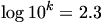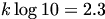Bear with me, I need to work on this a bit. Is the base 10 or something else (if one is given)?

Edit: If no bases are given, I'll assume the answer is:### #8jambo david

• Gender:Male

Posted 28 March 2006 - 07:55 PM

Ah, in that case, you use the laws of logarithms to simplify the expression:Bear with me, I need to work on this a bit. Is the base 10 or something else (if one is given)?

Edit: If no bases are given, I'll assume the answer is:the base isn't given

i really appreciate the help

### #9The Wedge Effect

HSN Legend

•• Members
•• 2,477 posts
• Location:Paisley
• Gender:Male

Posted 28 March 2006 - 07:56 PM

You're welcome. I wouldn't rely on my answer for k though, I'm not sure if it's correct.### #10SncZ

• Location:Edinburgh
• Gender:Male

Posted 28 March 2006 - 08:02 PM

thanks wedge,

for number 1, if there is no 'to the base of' we just got told to assume it's 1

log110k =2.3

12.3 =10k
1 =10k
log101 = log1010k
log101 = k (log1010)
log101 = k
k = 0

im not sure if thats right.. have a feeling its not, but its the best i can do at the moment

thats what i was told anyway (about the bases), im not trying to say wedge is wrong!

### #11George

Child Prodigy

••• 720 posts
• Location:West Lothian
• Gender:Male

Posted 28 March 2006 - 09:10 PM

for number 1, if there is no 'to the base of' we just got told to assume it's 1

That seems really odd - usually "log" is taken to mean either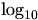or.

In fact, I don't think you can even have a logarithm with base 1! Here's why: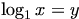means y is the power of 1 that gives x. Because 1 to any power is 1, you'll have trouble finding y if x isn't 1!

If your teacher has really told you to use log1, you should ask them about it (and then explain it to me)

for number 1, if there is no 'to the base of' we just got told to assume it's 1

log110k =2.3

12.3 =10k
1 =10k
log101 = log1010k
log101 = k (log1010)
log101 = k
k = 0

im not sure if thats right.. have a feeling its not, but its the best i can do at the moment

thats what i was told anyway (about the bases), im not trying to say wedge is wrong!

Your feeling is right, unfortunatelyIf you try k=0, you get.

Wedge's working is certainly correct, and it's as far as you can really take it.

#### 1 user(s) are reading this topic

0 members, 1 guests, 0 anonymous users